Average true range (ATR) is a technical analysis volatility indicator originally developed by J. Welles Wilder, Jr. for commodities. The indicator does not provide an indication of price trend, simply the degree of price volatility
(by Wikipedia)

Introduction

In this article, we suggest that there is some correlation between the strength of price trend and Average True Range Indicators. We suspect that when that ATR goes down, then a price trend is likely to the end. And we describes that by 3 chapters in this paper.
1. How To Calculate ATR? Describes a more details of ATR Indicator which was used for this research.
2. Chart Pattern Analysis. This is an abstract explanation by standard chart patterns. Sections: Double Top, Triangle.
3. Back Test Analysis. This is a concrete explanation by the 759679 orders of 5 currency pairs back-test result(2004 - 2014). Sections: Trading rule, Filters, EUR/USD(152304). GBP/USD(149175), USD/JPY(152798), USD/CHF(150498), USD/CAD(154904), Summary.
Chapter 1: How To Calculate ATR?

At first, you needs to calculate a true range. The range of a day's trading is a high minus low price. And the true range extends it to yesterday's closing price if it was outside of today's range. Next, calculate an N-day exponential moving average of the true range values. Calculation formula is as below.
• True Range = max(High, Previous Close) - min(Low, Previous Close).
• ATR = N-day exponential moving average of the TR.
Dukascopy's default ATR(unstable) is just like as above. However, I used an another ATR(stable) instead of that for this research. Because it was scored the better result. Of course it is likely just a matter of taste. So if you likes the default ATR(exponential moving) better than the ATR(simple moving), please use a your favorite one.
FYI: ATR(stable) is an N-day simple moving average of the true range values. If you want to get a sample indicator of ATR(stable), please search "ATRs" on the JStore.

Chapter 2: Chart Pattern Analysis.

In this chapter, we intend to the check whether there is some relationships between ATR and standard chart patterns. In this time, we use 2 chart patterns, Double Top and Triangle.

Double Top: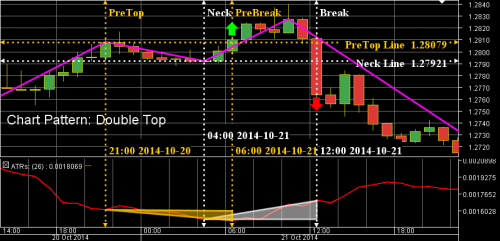Figure 2.1: Double top pattern

Figure 2.1 displays the Double Top Chart Pattern sample. Vertical lines are as below.
• PreTop is the time of previous top candle.
• PreBreak is the time of candle which break the close price of PreTop candle.
• Neck is the time of candle which has lowest close price between PreTop and Top.
• Break is time of candle which break the close price of Neck.
Check Points:
1. When ATR of the PreBreak point was less than that of PreTop Point, price trend direction was turned.
2. Contrary to this, when ATR value of the Break point was bigger than that of Neck point, price trend direction was keep a direction.
Triangle;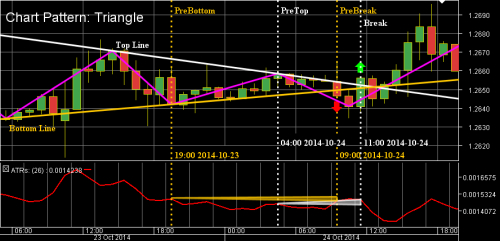Figure 2.2: Triangle pattern

Figure 2.2 displays the Triangle Chart Pattern sample. Vertical lines are as below.
• PreBottom is time of previous bottom candle.
• PreTop is time of previous top candle.
• PreBreak is time of candle which break the bottom line.
• Break is time of candle which break the top line. Check Points:
Check Points:
1. When ATR trend of the PreBreak point was less than that of PreBottom point, price trend was failed to go out from the triangle.
2. Contrary to this, when ATR trend of the Break point was bigger than that of PreTop point, price trend was succeed to go out from the triangle.
Chapter 3: Back Test Analysis

Next, in this chapter, we do explanation about a relationship between ATR and average gain pips by using a sample strategy.

Trading rules of sample strategy are as below.
• When last bar's close price was highest value of the last 26 bars should try to take a SHORT position.
• When last bar's close price was lowest value of the last 26 bars should try to take a LONG position.
• Stop loss line is +-0.5% of close price.
• Take profit line is +-0.1% of close price.
• All orders are closed at Friday 23:55 EET.
• Here is more detail, See the chapter2.
Filters:
Explanations of filters are as below.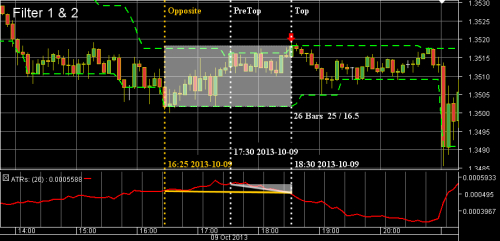Figure 3.1: Filter1 and Filter2

Figure 3.1 describes filtering pattern 1 and 2.
Vertical lines:
• Top is the time of candle which was send a sell signal.
• PreTop is the time of candle which is a second highest close price in last 26 bars.
• Opposite is the time of candle which has a lowest close price in last 26 bars.
Filter 1: ATR Value of Top line's candle is less than that of PreTop line's candle.
Filter 2: ATR Value of Top line's is less than that of Opposite line's candle.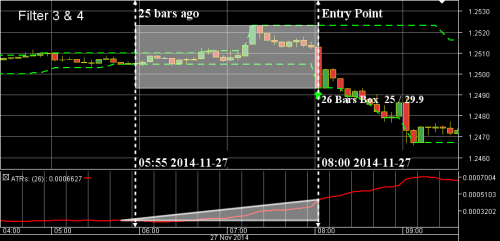Figure 3.2: Filter3 and Filter4

Figure 3.2 describes filtering pattern 3 and 4.
Vertical lines:
• Bottom is the time of candle which was send a buy signal.
• 25 bars ago is the time of candle which is 25 bars ago from Bottom.
Filtering 3: ATR Value of Bottom line's candle is highest in the last 26 bars.
Filtering 4: ATR Value of Bottom line's candle is more than that value of candle which is 25 bars ago from that candle.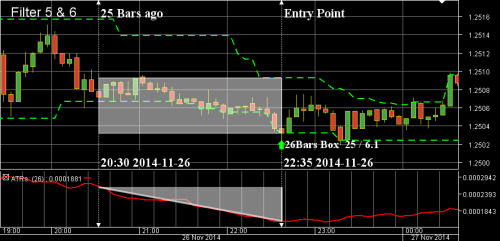Figure 3.3: Filter5 and Filter6

Figure 3.3 describes filtering pattern 5 and 6.
Vertical lines:
• Bottom is the time of candle which was send a buy signal.
• 25 bars ago is the time of candle which is 25 bars ago from Bottom.
Filter 5: ATR Value of Bottom line's candle is less than that value of candle which is 25 bars ago from that candle.
Filter 6: ATR Value of Bottom line's candle is lowest in the last 26 bars.Figure 3.4: Filter7 and Filter8

Figure 3.4 describes filtering pattern 7 and 8.
Vertical lines:
• Bottom is the time of candle which was send a buy signal.
• Opposite is the time of candle which has a highest close price in last 26 bars from Bottom.
• 25 bars ago is the time of candle which is 25 bars ago from Opposite.
Filter 7: ATR Value of Opposite line's candle is highest in the last 26 bars from that candle.
Filter 8: ATR Value of Opposite line's candle is more than that value of candle which is 25 bars ago from that candle.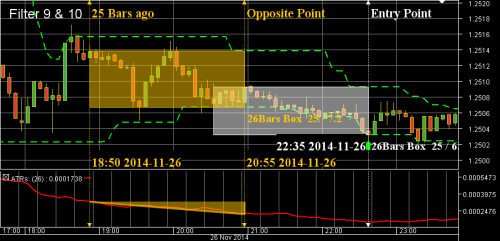Figure 3.5: Filter9 and Filter10

Figure 3.5 describes filtering pattern 9 and 10.
Vertical lines:
• Bottom is the time of candle which was send a buy signal.
• Opposite is the time of candle which has a highest close price in last 26 bars from Bottom.
• 25 bars ago is the time of candle which is 25 bars ago from Opposite.
Filtering 9: ATR Value of Opposite line's candle is less than that value of candle which is 25 bars ago from that candle.
Filtering 10: ATR Value of Opposite line's candle is lowest in the last 26 bars from that candle.

The following sectors summarizes filtering result of the above descriptions.

EUR/USD: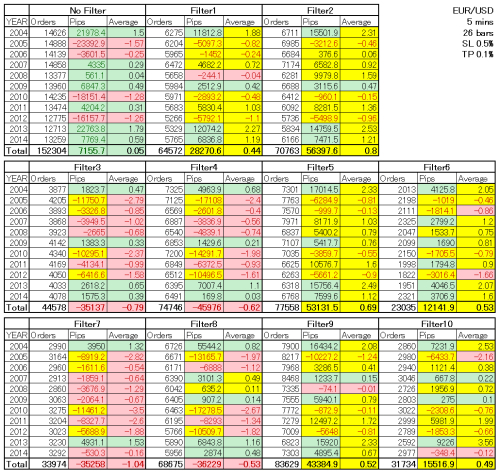Table 1: EUR/USD-Summary of back test result filtering. *Background Color: Light Green is a positive integers, Light Red is a negative integers, Yellow is greater than default value. Text Color: Black is a positive integers, Dark Red is a negative integers*Figure 3.61: EUR/USD-Average of gain pips before and after filtering

Point: Average gain pips which are after filtering by filter 1, 2, 5, 6, 9, or 10 are better than before filtering. On the other hand, that of after filtering by filter 3, 4, 7, or 9 are worse than before that. And this result shows us that counter strategy is strong in the ATR downtrend situation.

GBP/USD:Table 2: GBP/USD-Summary of back test result filtering.Figure 3.62: GBP/USD-Average gain pips before and after filtering

Point: Same as EUR/USD, this result shows us that counter strategy is strong in the ATR downtrend situation.

USD/JPY:Table 3: USD/JPY-Summary of back test result filtering.Figure 3.63: USD/JPY-Average gain pips before and after filtering

Point: Filter 10 was good. But, filter 6 was worst, and it was very surprised me. We have to have some special caution to this pair. And this pair require us to check the order time(see this articles).

USD/CHF: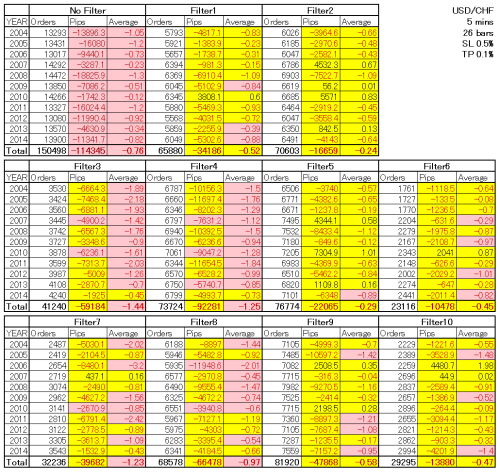Table 4: USD/CHF-Summary of back test result filtering.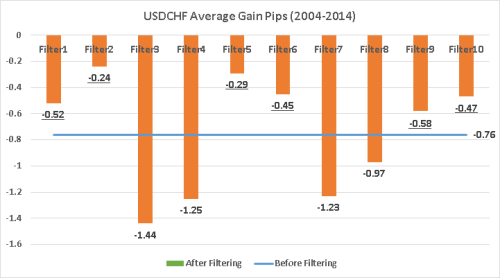Figure 3.64: USD/CHF-Average gain pips before and after filtering

Point: Same as EUR/USD and GB/PUSD, this result shows us that counter strategy is strong in the ATR downtrend situation.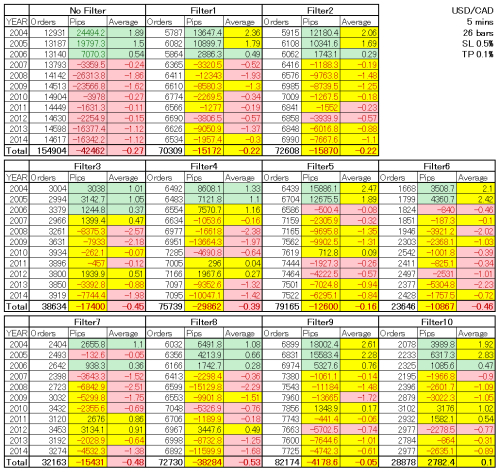Table 5: USD/CAD-Summary of back test result filtering.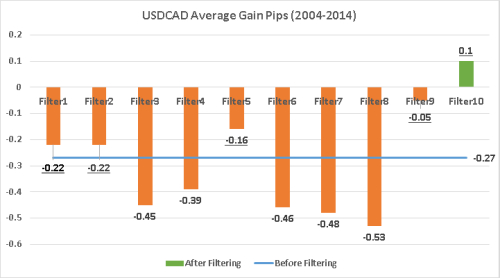Figure 3.65: USD/CAD-Average gain pips before and after filtering